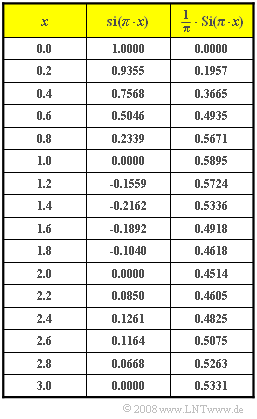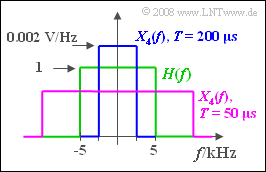# Exercise 1.5: Rectangular-in-Frequency Low-Pass FilterTable with values of the  $\rm si$–function and the  $\rm Si$–function

We consider an ideal, rectangular low-pass filter – also called a Küpfmüller low-pass filter,

• which passes all frequencies  $f < 5 \ \rm kHz$  in an undistorted way   ⇒   $H(f) = 1$,
• and completely suppresses all spectral components above  $5 \ \rm kHz$    ⇒   $H(f) = 0$.

Exactly at the cut-off frequency  $f_{\rm G} = 5 \ \rm kHz$  the value of the transfer function is equal to  $1/2$.

Various signals are applied to the input of the low-pass filter:

• a narrow rectangular pulse of suitable height which can be approximated by a "Dirac":
$$x_1(t) = 10^{-3}\hspace{0.1cm}{\rm Vs} \cdot {\rm \delta}(t),$$
• a Dirac pulse, i.e., a sum of Dirac functions evaluated at the respective time interval of  $T_{\rm A}$:
$$x_2(t) = 10^{-3}\hspace{0.1cm}{\rm Vs} \cdot \sum_{\nu = -\infty}^{+\infty}{\rm \delta}(t - \nu \cdot T_{\rm A}),$$
where the associated spectrum with  $f_{\rm A} = 1/T_{\rm A}$  is:
$$X_2(f) = \frac{10^{-3}\hspace{0.1cm}{\rm Vs}}{T_{\rm A}} \cdot\sum_{\mu = -\infty}^{+\infty}{\rm \delta}(f - \mu \cdot f_{\rm A}),$$
• a step function at time  $t = 0$:
$$x_3(t) = 10\hspace{0.1cm}{\rm V} \cdot \gamma(t) = \left\{ \begin{array}{c} 0 \\ 5\hspace{0.1cm}{\rm V} \\ 10\hspace{0.1cm}{\rm V} \\ \end{array} \right.\quad \quad\begin{array}{*{10}c} {\rm{f\ddot{u}r}} \\ {\rm{f\ddot{u}r}}\\ {\rm{f\ddot{u}r}} \\ \end{array}\begin{array}{*{20}c}{ t < 0,} \\{ t = 0,} \\ { t > 0,} \\ \end{array}$$
• an  $\rm si$–shaped pulse with equivalent duration  $T$:
$$x_4(t) = 10\hspace{0.1cm}{\rm V} \cdot {\rm si}(\pi \cdot {t}/{T}) \hspace{0.5cm} {\rm mit} \hspace{0.5cm} {\rm si}(x) = {\rm sin}(x)/x .$$

• The exercise belongs to the chapter  Some Low-Pass Functions in Systems Theory.
• The table lists the function values of the sinc function  ${\rm si}(πx)$ and the sine integral function  ${\rm Si}(πx)$:
$${\rm Si}(\pi x) = \int_{ 0 }^{ x } {{\rm si} ( \pi \xi )} \hspace{0.1cm}{\rm d}\xi \hspace{0.5cm}{\rm mit } \hspace{0.5cm} {\rm si}(x) =\sin(x)/x.$$

### Questions

1

What is the output signal  $y_1(t)$  in response to the Dirac function  $x_1(t)$, in particular at times  $t = 0$  and  $t = 50 \ \rm µ s$?

 $y_1(t = 0) \ = \$  $\rm V$ $y_1(t = 50 {\: \rm µ s}) \ = \$  $\rm V$

2

What is the output signal  $y_2(t)$, if the dirac pulse  $x_2(t)$  is applied to the filter input and  $T_{\rm A} = 200 \ µ \rm s$  holds. What signal value occurs at  $t = 0$ ?

 $y_2(t = 0) \ = \$  $\rm V$

3

What values  $y_2(t = 0)$  are obtained with  $T_{\rm A} = 199 \ \rm µ s$  or  $T_{\rm A} = 201 \ \rm µ s$?

 $T_{\rm A} = 199 \ {\rm µ s} \text{:}\hspace{0.4cm} y_2(t = 0) \ = \$  $\rm V$ $T_{\rm A} = 201 \ {\rm µ s} \text{:}\hspace{0.4cm} y_2(t = 0) \ = \$  $\rm V$

4

State the output signal  $y_3(t)$  for the step function  $x_3(t)$  with final value  $10 \ \rm V$ . What is the signal value at time  $t = 0$ ?

 $y_3(t = 0) \ = \$  $\rm V$

5

At what time  $t_{\rm max}$  does  $y_3(t)$  attain its maximum value? What is the maximum value??

 $t_{\rm max} \ = \$  $\rm µ s$ $y_3(t_{\rm max}) \ = \$  $\rm V$

6

What is the output signal  $y_4(t)$, if the  ${\rm si}$–shaped signal  $x_4(t)$  with  ${\rm si}(πx)$ $T = 200 \ \rm µ s$  is applied to the input? What value is obtained for  $t = 0$?

 $y_4(t = 0) \ = \$  $\rm V$

7

What signal value  $y_4(t = 0)$  is obtained for  $T = 50 \ \rm µ s$?

 $y_4(t = 0) \ = \$  $\rm V$

### Solution

#### Solution

(1)  With   $Δf = 10 \ \rm kHz$, the impulse response of the ideal low-pass filter is given by:

$$h(t) = \Delta f \cdot {\rm si}(\pi \cdot \Delta f \cdot t ).$$
• The output signal differs from this by the weighting factor  $\rm 10^{–3} \ \rm Vs$:
$$y_1(t) = 10^{-3}\hspace{0.1cm}{\rm Vs} \cdot 10^{4}\hspace{0.1cm}{\rm Hz} \cdot {\rm si}(\pi \cdot \Delta f \cdot t ) = 10\hspace{0.1cm}{\rm V} \cdot {\rm si}(\pi \cdot \Delta f \cdot t )$$
$$\Rightarrow \hspace{0.2cm}y_1(t = 0) \hspace{0.15cm}\underline{=10\hspace{0.1cm}{\rm V}},\hspace{0.5cm}y_1(t = 50\hspace{0.1cm}{\rm µ s}) =10\hspace{0.1cm}{\rm V} \cdot {\rm si} \left( {\pi}/{2} \right) \hspace{0.15cm}\underline{= 6.37\hspace{0.1cm}{\rm V}}.$$

(2)  The spectrum  $X_2(f)$  contains discrete lines at an interval of  $f_{\rm A} = 1/T_{\rm A} = 5 \ \rm kHz$, each with weight  $5 \ \rm V$.

• The spectrum  $Y_2(f)$  thus consists of one spectral line at  $f = 0$  with weight  $5 \ \rm V$  and one each at  $±5 \ \rm kHz$  with weight  $2.5 \ \rm V$. Hence, the following is true for the time signal:
$$y_2(t) = 5 \hspace{0.1cm}{\rm V} + 5\hspace{0.1cm}{\rm V} \cdot{\rm cos}(2 \pi \cdot f_{\rm A} \cdot t ) = 10\hspace{0.1cm}{\rm V} \cdot {\rm cos}^2(\pi \cdot f_{\rm A} \cdot t ).$$
$$\Rightarrow \hspace{0.3cm} y_2(t= 0) \hspace{0.15cm} \underline{ = 10\hspace{0.1cm}{\rm V}} .$$

(3)  Due to  $T_{\rm A} = 199 \ \rm µ s$ ,   $f_{\rm A} > 5 \ \rm kHz$ holds. Because of  $H(f_{\rm A}) = 0$  the spectrum consists of only one spectral line at  $f = 0$  with weight  $5.025 \ \rm V$ and the following constant curve is obtained

$$\Rightarrow \hspace{0.3cm} y_2(t) \rm \underline{\: = 5.025 \ \rm V}.$$
• If  $T_{\rm A}$  is further decreased, the output still yields a direct signal but with larger signal value (proportional to $1/T_{\rm A}$).
• In contrast to this, with  $T_{\rm A} = 201 \ \rm µ s$  the sampling frequency is slightly smaller than the cut-off frequency of the filter  $(5 \ \rm kHz)$ and the spectral function of the output signal is:
$$Y_2(f) = 4.975\hspace{0.1cm}{\rm V} \cdot \big[ {\rm \delta}(f ) + {\rm \delta}(f + f_{\rm A}) + {\rm \delta}(f - f_{\rm A})\big].$$
• From this it follows for the time signal:
$$y_2(t ) = 4.975\hspace{0.1cm}{\rm V} + 9.95\hspace{0.1cm}{\rm V} \cdot {\rm cos}(2 \pi \cdot f_{\rm A} \cdot t ) \hspace{0.2cm} \Rightarrow \hspace{0.2cm}y_2(t = 0) \hspace{0.15cm}\underline{=14.925\hspace{0.1cm}{\rm V}}.$$
• Nothing changes in the fundamental curve as long as  $200 \ {\rm µ s} < T_{\rm A} < 400 \ {\rm µ s}$  holds.
• However, different amplitudes arise as a result depending on  $T_{\rm A}$ .   For  $T_{\rm A} ≥ 400 \ {\rm µ s}$ additional spectral lines are added.

(4)  The output signal  $y_3(t)$  now goes according to the sine integral function:

$$y_3(t = 0) = 10\hspace{0.1cm}{\rm V} \cdot \Delta f \cdot \int_{ - \infty }^{ t } \hspace{-0.3cm} {{\rm si} ( \pi \Delta f \tau )} \hspace{0.1cm}{\rm d}\tau = 10\hspace{0.1cm}{\rm V} \cdot \big[ {1}/{2} + {1}/{\pi}\cdot {\rm Si} ( \pi \Delta f t )\big].$$
$$\Rightarrow \hspace{0.3cm} y_3(t= 0) \hspace{0.15cm} \underline{ = 5\hspace{0.1cm}{\rm V}} .$$

(5)  It is obvious that  $y_3(t)$  attains its maximum when the  ${\rm si}$–function intersects the abscissa for the first time at positive times (see sketch). So, it must hold::

$$t_{\rm max} = 1/Δf \rm \underline{\: = 100 \: µ s}.$$
• The signal value is obtained according to the table on the information page and is
$$y_3(t = t_{\rm max}) = 10\hspace{0.1cm}{\rm V} \cdot \big[ {1}/{2} + {1}/{\pi}\cdot {\rm Si} ( \pi )\big]= 10\hspace{0.1cm}{\rm V} \cdot \big[ 0.5 + 0.5895 \big] \hspace{0.15cm}\underline{= 10.895 \hspace{0.1cm}{\rm V}} .$$
• At later times  $t$ ,   $y_3(t)$  slowly adjusts to its final value  $10 \ \rm V$.Rectangular spectra at the input of the rectangular filter

(6)  The spectral function  $X_4(f)$  is rectangular like  $H(f)$  and for  $|f| > 2.5 \ \rm kHz$  always zero.

• This means that  $Y_4(f ) = X_4(f)$  holds and so does  $y_4(t) = x_4(t)$.
• Thus,  $y_4(t = 0) \rm \underline{\: = 10 \: \rm V}$.

(7)  Due to  $T = 50 \ \rm µ s$ ,   $X_4(f)$  has the width  $20 \ \rm kHz$  and the height  $0.5 · 10 ^{-3} \ \rm V/Hz$.

• The spectral function  $Y_4(f)$ has the same height   after being multiplied by  $H(f)$ .
• The width  $10 \ \rm kHz$  is now determined exclusively by  $H(f)$ :
$$y_4(t) = 0.5 \cdot 10^{-3}\hspace{0.1cm}{ {\rm V} }/{ {\rm Hz} } \cdot 10 \hspace{0.1cm}{\rm kHz} \cdot {\rm si}(\pi \Delta f t ) = 5\hspace{0.1cm}{\rm V} \cdot {\rm si}(\pi \Delta f t )$$
$$\Rightarrow \hspace{0.3cm}y_4(t = 0) \hspace{0.15cm}\underline{=5\hspace{0.1cm}{\rm V} }.$$